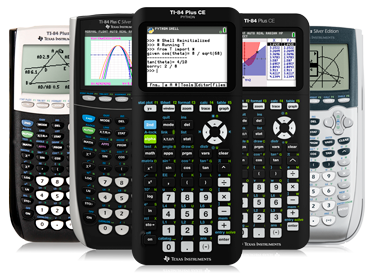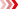Find activities that support your lesson plans

Challenge students to use and think about how technology can be used to model, analyze and explain math with standards-aligned activities in six middle grades math topics.

Activities can be edited or used as is, giving you the flexibility to use them to support specific objectives, and include technology tips to help you focus the learning and address student misconceptions.# Featured Activities

## Transforming Fish

In this activity, students will discover how pictures formed by graphing ordered pairs can be stretched (and shrunk) by multiplying (and dividing) the coordinates.## The Variables of Renting

In this activity, students will identify what a variable is, construct a table of values, graph the ordered pairs from the table, and graph the equation.## Roll the Dice

In this activity, students compute sample spaces for coin flips, find the various probabilities associated, and graph the probabilities using the calculator.